# The Complete Guide to Data Structures with JavaScriptIn this article, we will explains different parts of Data Structures With JavaScript in a very simple and straightforward way.

In this article, we will explains different parts of Data Structures With JavaScript in a very simple and straightforward way.

“The Complete Guide to Data Structures with JavaScript” that explains different parts of Data Structures with JavaScript in a very simple and straightforward way. Here are the parts of the article:

1. Creating 3 Stacks With 1 Array in JavaScript
2. Stacks vs. Queues In JavaScript
3. Creating Linked Lists With JavaScript
4. Creating Graphs With JavaScript

## Creating 3 Stacks With 1 Array in JavaScript

### What Is A Stack?

A stack is a data structure that is based on the concept of “last-in-first-out” or “LIFO.” You can think of it like a stack of books where the top book has to be taken off the stack before you can retrieve the bottom book. JavaScript doesn’t have a native stack data structure, so we’re going to create one today.

Our array will contain three different stacks of a fixed size. The top of the stack will be on the right side and the bottom of the stack will be on the left side. You can picture it similar to this diagram. If this stack was full, the bottom element would live at `stack` and the top element would live at `stack[stack.length-1]`.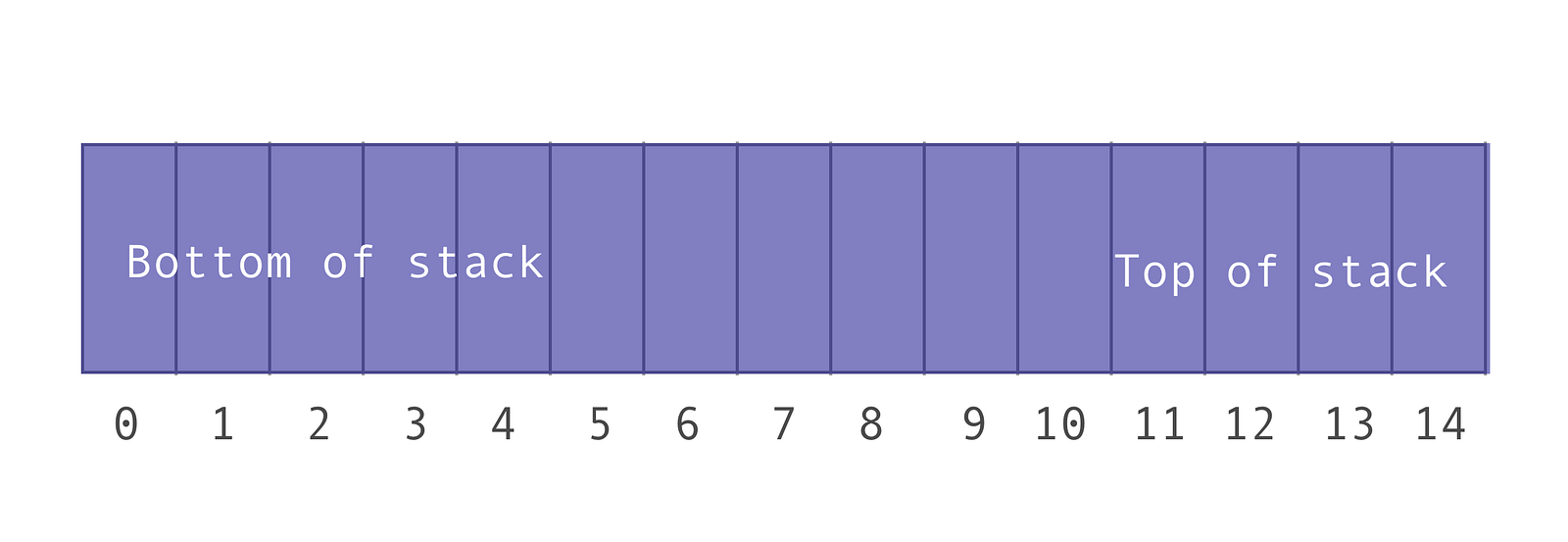### Class Breakdown

Our stacks will have a fixed size which will be equal to the argument passed in at instantiation.

#### Properties

The following properties will be initialized within the constructor:

• `_stackCapacity`: The maximum number of elements that can fit into one stack. This is a read-only property which is why it’s prepended with an underscore.
• `values`: An array which contains of all elements in the three stacks
• `sizes`: An array with three indices, each representing the current number of elements in the respective stacks.
• `numberOfStack`s: A constant which represents the total number of stacks we’ll allow our array to hold. We’re initializing this to three, however future iterations of this MultiStack class could take in a second argument to customize the number of stacks the array can hold.

#### Methods

Our MultiStack class will contain the following methods:

• `get stackCapacity()`: Returns the total capacity of each of the stacks (this is just a way for me to check that everything is working as expected, we won’t really be using this.)
• `push(stackNumber, value)`: Pushes the value onto the top of the respective stack number.
• `pop(stackNumber)`: Pops the top item off of the respective stack number.
• `peek(stackNumber)`: Returns the top item off of the respective stack number. It’s just a way for us to “peek” at what element is on the top; no stack mutation will happen.
• `isEmpty(stackNumber)`: Returns a boolean which indicates whether the respective stack has values.
• `isFull(stackNumber)`: Returns a boolean which indicates whether the respective stack is full.
• `indexOfTop(stackNumber)`: A helper method which returns the index, in the values array, of the top element in the respective stack.

#### Constructor

The first thing we’ll do is create our constructor. It will take in one argument, the stack size. Thus, the total length of our values array will be 3 * the stack size (since we’re initializing `numberOfStacks` to three).

We will initialize the sizes array to contain three indices with the value zero. For our purposes we will assume that the values being pushed onto the stacks are positive integers. You can change this logic to fit your needs.

#### Get Stack Capacity

This method returns the total capacity of each of the stacks (this is just a way for me to check that everything is working as expected, we won’t really be using this.)

#### isFull

This method returns a boolean which indicates whether the respective stack is full. It will check how many elements are currently on the respective stack and compare it against the stack capacity.

#### isEmpty

This method returns a boolean which indicates whether the respective stack has values.

#### indexOfTop

This is a helper method which returns the index, in the values array, of the top element in the respective stack.

This explanation could get a little tricky, so stick with it! I’ve included diagrams to better visualize the process.

First we need to grab the offset of the stack within the values array. To do this, we’ll multiply the stack number we want by the capacity of each stack.

For example, let’s find the index of the top item in stack 2 given that the `_stackCapacity` for each stack is 5. The stacks contain the following elements:

• Stack 0: [1, 12]
• Stack 1: [18, 8, 2]
• Stack 2: [5, 9, 66, 15]

Here is a visual representation of what the values array looks like:

Step 1: Calculate the offset; find the index of the bottom item in stack two

Assuming our stacks start at zero (i.e. stack 0, stack 1, stack 2), we can find where the bottom of stack two starts in the values array by multiplying the stack we’re looking for, two, by the stack capacity, which is the value passed in at instantiation. If our stack capacity is five, we know that the bottom element of stack two starts at index 10 in the values array.

index of bottom element in stack 2 = stack we’re looking for * capacity of each stack.

index of bottom element in stack 2 = 2 * 5 (found from `_stackCapacity`)

index of bottom element in stack 2 = 10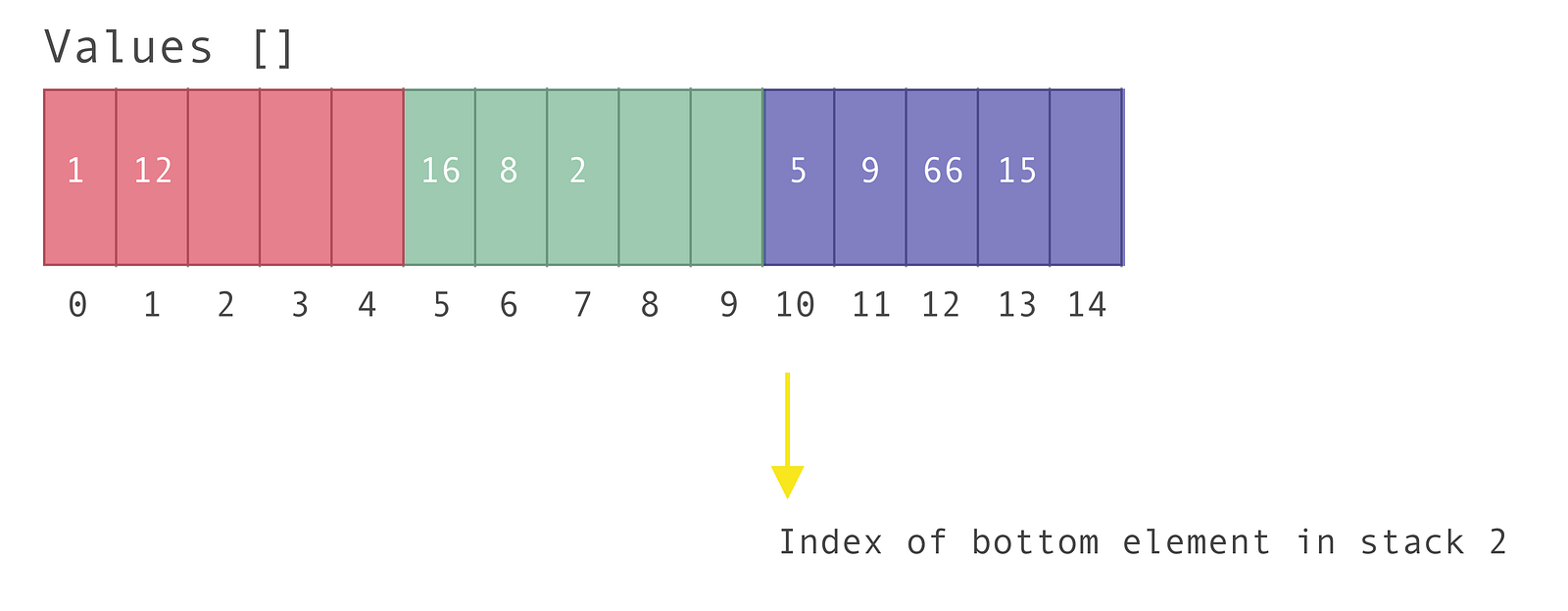Step 2: Calculate the total number of values currently in stack two

We already know how many values are in stack 2; they’re being kept in the sizes array. So by grabbing the value of `sizes` we know how many elements are in stack 2: 4

Step 3: Add the offset with the total number of values in the stack, minus one

We have to subtract one from the number of items in the stack, since our array starts at index zero.

When we add it all up we get:

index of top element in stack 2 = offset + number of values in stack two — 1

index of top element in stack 2 = 10 + 4 — 1

index of top element in stack 2 = 13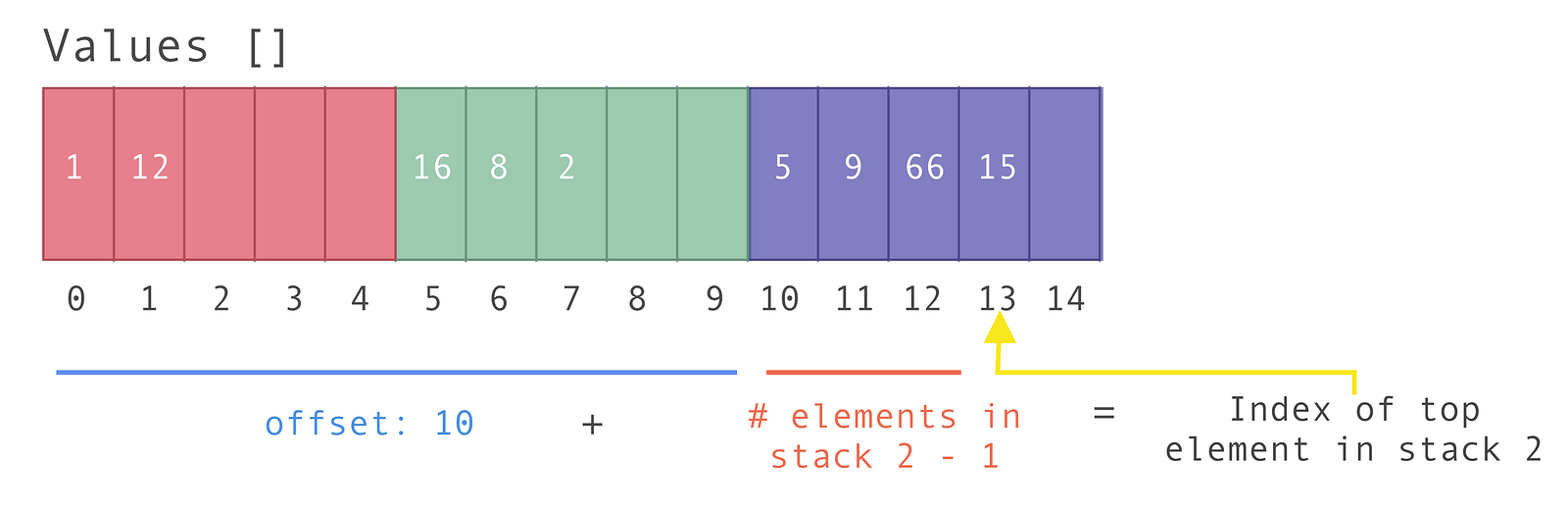The code for this is as follows:

#### Push

The push method pushes a value onto the top of the respective stack. It takes in two arguments:

• The stack to push the value onto
• The value
1. The first thing we have to do is check whether the stack is full. If it is full, let’s `console.log` the message `Stack number \${stackNumber} is full`.
2. If the stack isn’t full, increase the number of items in the stack, which is found in the sizes array.
3. Then add the new value to the top of the stack. We’ll use the `indexOfTop` method we just explained above to grab the top of the stack and add a value on top of it.
4. If it’s successfully added, let’s `console.log` a friendly message.

#### Pop

This method pops the top item off of the respective stack number. It takes in one argument:

• The stack to pop the value off of
1. We must first check if the stack is empty using the `isEmpty` method. If it is, we’ll return a `console.log` a message.
2. If the stack isn’t empty, we’ll grab the index of the top element on the stack using the `indexOfTop` method and save it to a variable called `topIndex`.
3. Now let’s grab the value of that element. We can do this with `this.values[topIndex]` . We’ll return this element, which is why we need to save it to a variable.
4. We also need to tell the values array that the value at this index no longer exists. We’ll set this explicitly to zero (this could pose issues if your stack can take zero as a value, but for our sake we’ll assume the stack only accepts positive integers).
5. We must also decrement the size of the stack in the sizes array. We can do this with `this.sizes[stackNumber]--`.
6. Finally, let’s return the value we just popped off.

#### Peek

This method returns the top item off of the respective stack number. It doesn’t alter the stack, it simply lets you view the element on the top. It takes in one argument:

• The stack whose top value we want to peek at
1. We first have to check if the stack is empty. We can use the isEmpty method to do so. If it is empty, let’s `console.log` a friendly message.
2. If the stack isn’t empty, we need to find the index for the element on top of the stack. We can use the `indexOfTop` method to do so.
3. Finally, we can return the value found at that index with `this.values[topIndex]`.

### Putting It All Together

The final class looks like this:

You’ve now created an array that represents three fixed-size stacks! You can view the CodePen:

See the Pen Creating 3 Stacks With 1 Array in JavaScript by Emma Wedekind (@emmawedekind) on CodePen.

## Stacks vs. Queues In JavaScript

Queues and stacks are two common data structures leveraged on technical interviews. Due to the fact that they’re quite similar in structure, they can be a bit confusing to differentiate. So today we’ll build a stack and a queue in JavaScript.

### Stacks

Stacks are data structures that follow the “last-in-first-out” or “LIFO” paradigm. We can think of them like a stack of books. In order to retrieve the third book in the stack, we have to take the fifth book off first, then the fourth book, until we retrieve the third book.

JavaScript doesn’t provide a native stack data structure, so we have to build our own with an array and a closure or a class.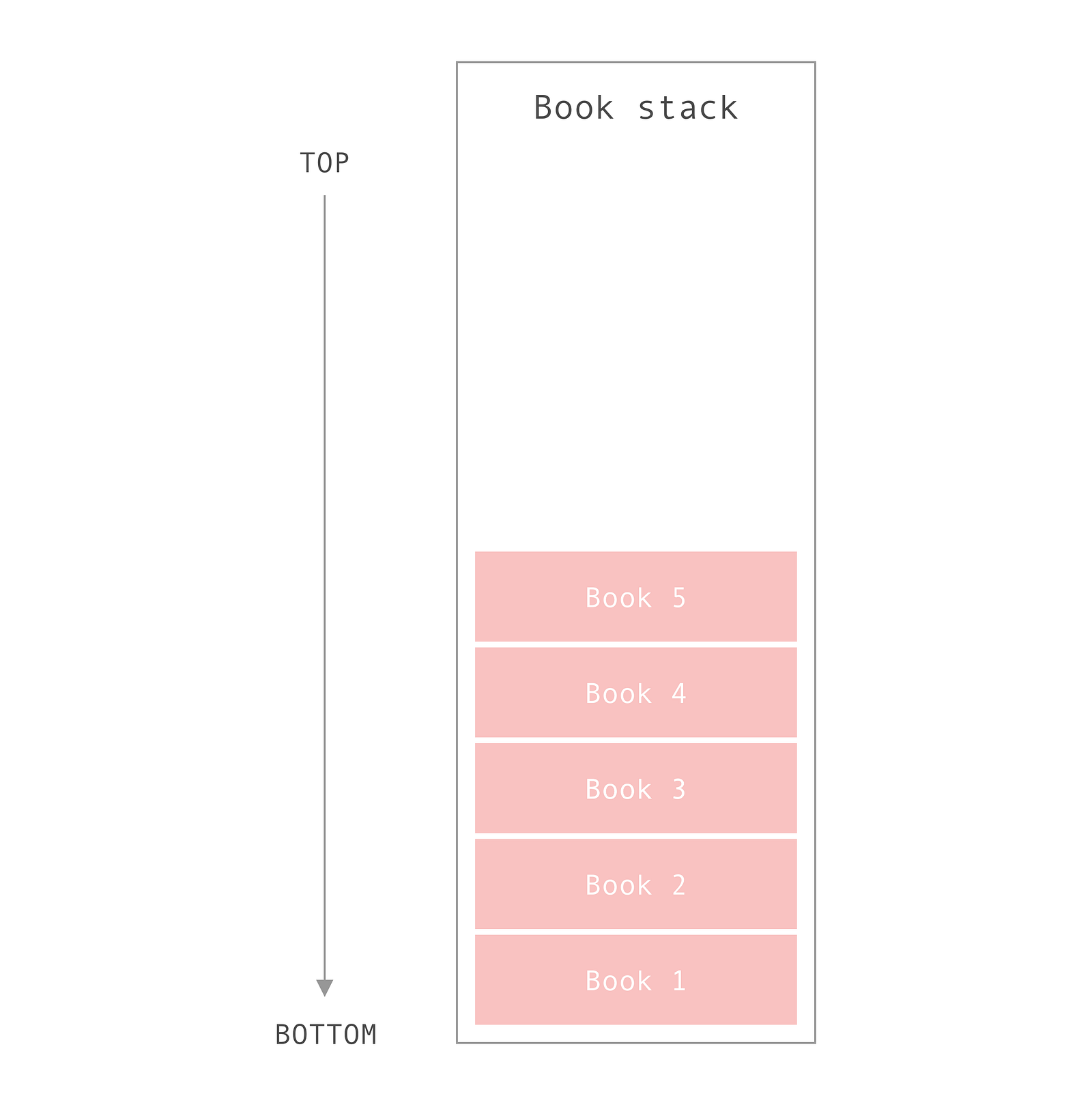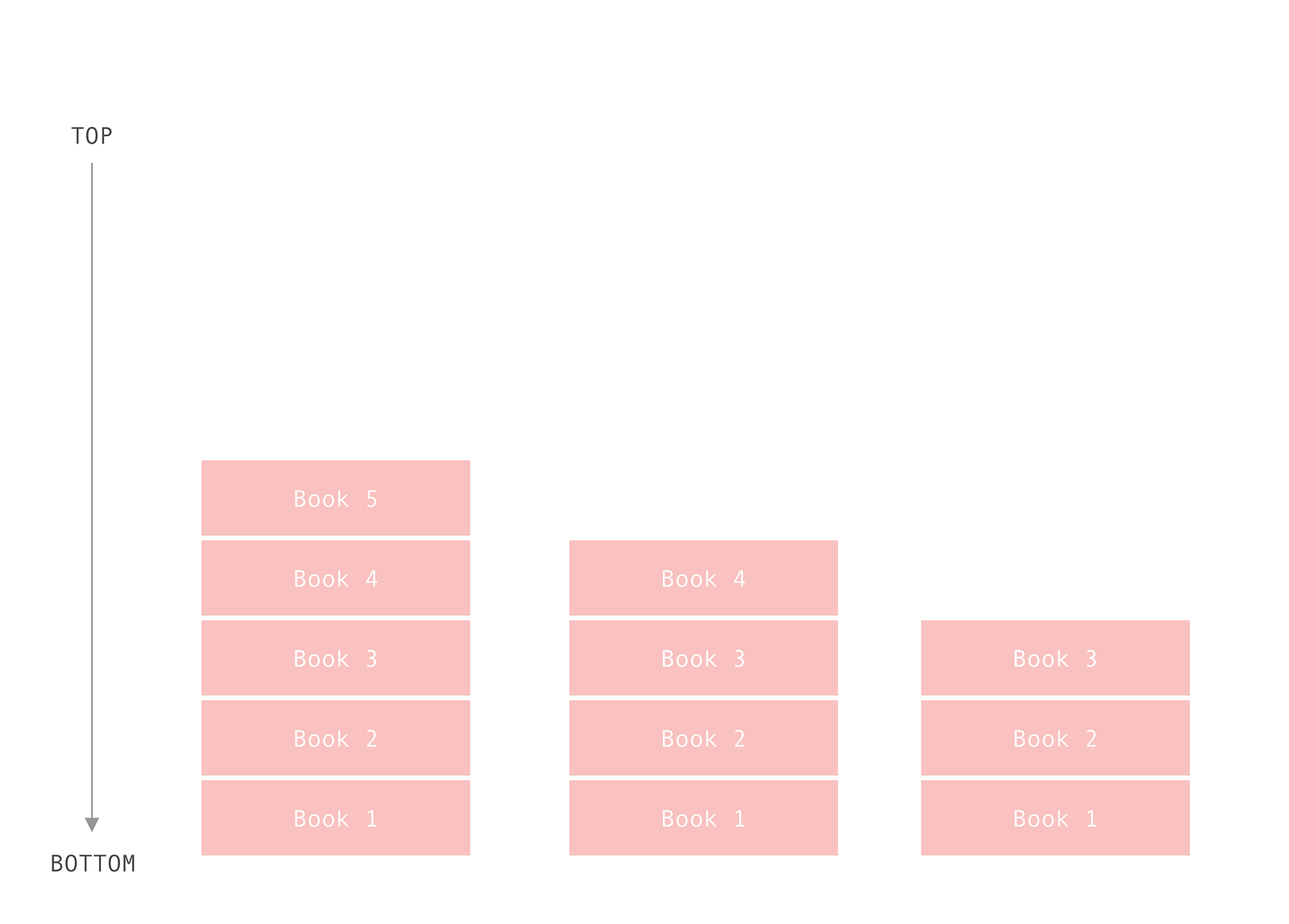#### Benefits

Stacks allow for constant-time adding and removing of an item. This is due to the fact that we don’t need to shift items around to add and remove them from the stack.

#### Constraints

Stacks, unfortunately, don’t offer constant-time access to the nth item in the stack, unlike an array. This means it can possible take O(n) where n is the number of elements in the stack, time to retrieve an item.

#### Methods

Stacks leverage the following methods:

• `pop()`: Remove the top item from the stack
• `push(item)`: Add an item to the top of the stack
• `peek()`: Return the item at the top of the stack
• `isEmpty()`: Returns true if the stack is empty

#### Let’s Build

Let’s build a BookStack which will contain a stack of our favorite novels. What’s great about stacks is that the push and pop methods are the same name as the corresponding array methods we’ll use.

Constructor

We’ll define a class BookStack and give it a constructor method that has one property:

• `this.stack = [];`
``````constructor() {
this.stack = [];
}
``````

Get

I’ll be adding a getter which returns the length of the stack. We’ll use this throughout our other methods.

``````get length() {
return this.stack.length;
}
``````

Push

We want to add the item to the end of the array, so we can use the `array.push()` method. The `array.push()` method returns the new length array.

``````push(item) {
return this.stack.push(item);
}
``````

Pop

We want to remove the last item in the array, so we can use the `array.pop()` method. The `array.pop()` method returns the item which was added, or undefined if the array is now empty.

``````pop() {
return this.stack.pop();
}
``````

Peek

We want to return, or peek at, the last item in the stack. Thus we just need to access the value at the last index.

``````peek() {
return this.stack[this.length - 1];
}
``````

isEmpty

We want to return true if there are no items in the stack. So if the length is zero, return true.

``````isEmpty() {
return this.length === 0;
}
``````

#### Putting It All Together

Our final BookStack code looks like this:

``````class BookStack {
constructor() {
this.stack = [];
}

push(item) {
return this.stack.push(item);
}

pop() {
return this.stack.pop();
}

peek() {
return this.stack[this.length - 1];
}

get length() {
return this.stack.length;
}

isEmpty() {
return this.length === 0;
}
}
``````

You can also create this with a closure.

``````function BookStack() {
const stack = [];

return {
push(item) {
return stack.push(item);
},

pop() {
return stack.pop();
},

peek() {
return stack[this.length - 1];
},

get length() {
return stack.length;
},

isEmpty() {
return this.length === 0;
}
}
}
``````

Let’s test it out with some book data.

``````let myBookStack = new BookStack();
myBookStack.push('Oathbringer');
myBookStack.push('The Stand');
console.log(myBookStack.length); // 2
console.log(myBookStack.peek()); // The Stand
myBookStack.pop();
console.log(myBookStack.length); // 1
console.log(myBookStack.peek()); // Oathbringer
console.log(myBookStack.isEmpty()); // false
myBookStack.pop();
console.log(myBookStack.isEmpty()); // true
``````

You can view the CodePen here.

### Queues

A queue is similar to a stack in structure and methods, however the paradigm is different. Queues use the “first-in-first-out” or “FIFO” method. This can be thought of like a queue, or line, of people waiting to buy movie tickets.

The person who’s been waiting the longest in line gets served before the person who just joined.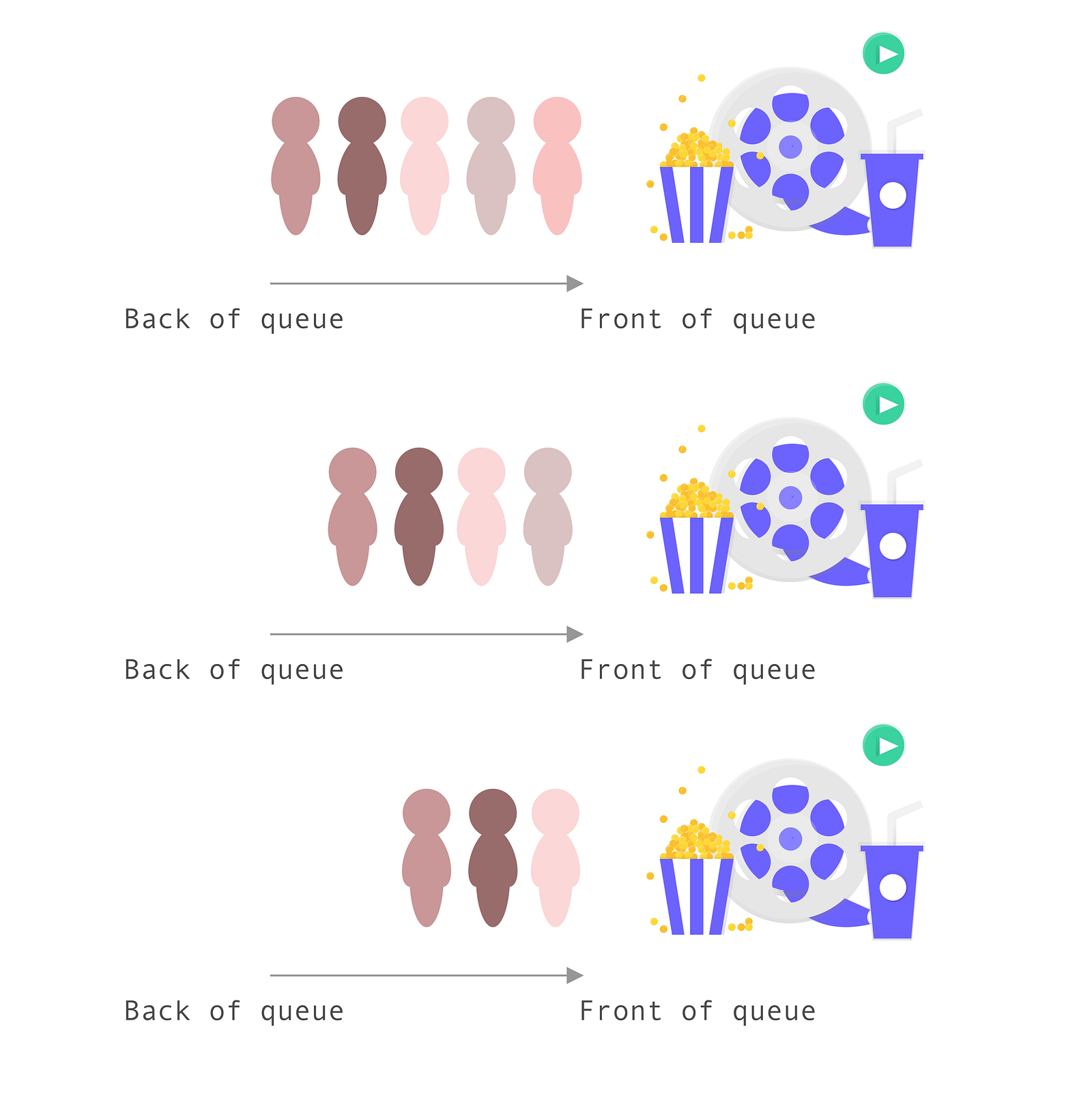#### Use Cases

Queues are very similar to linked lists and are typically used in breadth-first searches or when implementing a cache.

#### Constraints

Queues are much harder to update when adding and removing nodes.

#### Methods

Queues leverage the following methods:

• `enqueue(item)`: Remove the top item from the queue
• `dequeue()`: Add an item to the top of the queue
• `peek()`: Return the item at the top of the queue
• `isEmpty()`: Returns true if the queue is empty

#### Let’s Build

For this example, we’ll be using JavaScript classes. Please refer to the stack section if you’d like to see the function closure in action.

Constructor

We’ll define a class MovieQueue and give it a constructor method that has one property:

• `this.queue = [];`
``````constructor() {
this.queue = [];
}
``````

Get

I’ll be adding a getter which returns the length of the queue. We’ll use this throughout our other methods.

``````get length() {
return this.queue.length;
}
``````

Enqueue

We want to add an item to the first index in an array (the back of the queue). So let’s use the `array.unshift()` method.

``````enqueue(item) {
return queue.unshift(item);
}
``````

Dequeue

We want to remove the first item in the queue, or the last item in the array. We can simply use the `array.pop()` method to do this.

``````dequeue() {
return queue.pop();
}
``````

Peek

We want to see what the first item in the queue is. Remember this is the last item in the array. We’ll use `queue[this.length — 1]` to grab this value.

``````peek() {
return queue[this.length - 1];
}
``````

isEmpty

We want to return true if the queue is empty. We can use the length method to grab this information.

``````isEmpty() {
return this.length === 0;
}
``````

#### Putting It All Together

Our final MovieQueue code looks like this:

``````class MovieQueue {
constructor() {
this.queue = [];
}

enqueue(item) {
return this.queue.unshift(item);
}

dequeue() {
return this.queue.pop();
}

peek() {
return this.queue[this.length - 1];
}

get length() {
return this.queue.length;
}

isEmpty() {
return this.queue.length === 0;
}
}
``````

Let’s test it out with some names.

``````const myMovieQueue = new MovieQueue();
myMovieQueue.enqueue('Sandra');
myMovieQueue.enqueue('Rob');
myMovieQueue.enqueue('Lisa');
myMovieQueue.enqueue('Kai');
console.log(myMovieQueue.length); // 4
console.log(myMovieQueue.peek()); // Sandra
myMovieQueue.dequeue();
myMovieQueue.dequeue();
console.log(myMovieQueue.peek()); // Lisa
``````

You can view the CodePen:

See the Pen MovieQueue by Emma Wedekind (@emmawedekind) on CodePen.

## Creating Linked Lists With JavaScript

A singly linked list is a data structure which represents a series of nodes where each node points to the next node in the list. A doubly linked list, in contrast, has nodes which point to the element preceding and following it.

Unlike an array, a linked list doesn’t provide constant-time access to specific indices within the list. So if you need the third element in the list, you have to iterate past the first and second nodes to reach it.

One benefit of a linked list is the ability to add and remove items from the beginning and end of the list in constant time.

These are popular data structures to be questioned about during a technical interviews, so let’s jump right in.

A singly-linked list may be LIFO (last-in-first-out) or FIFO (first-in-first-out). If the list is using the LIFO method, the nodes will be added to and deleted from the same end. If it’s using FIFO, nodes will be added to one end and deleted from the opposite end.

Additionally, the linked list may be sorted. This means that as each node is added to the list, it’s placed into its appropriate spot relative to the other nodes.

### Node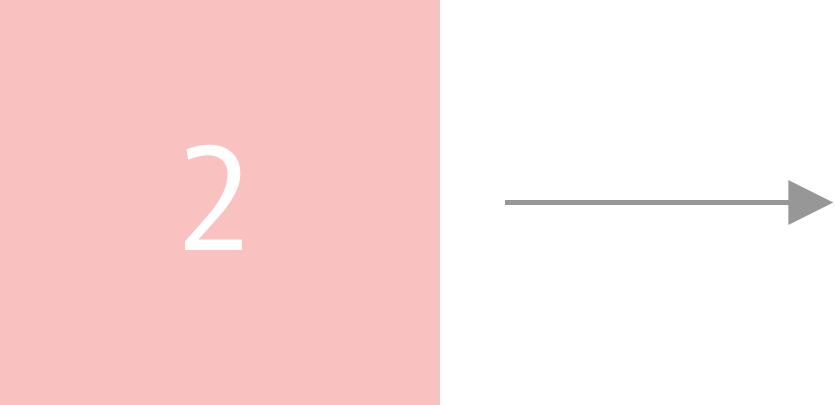A node has two pieces of information:

• A pointer, or reference, to the next item in the list (for a singly linked list)
• The value of the node

For our node, we’ll just create a function which takes a value, and returns an object with the two values above: a pointer to the next node and the value of the node. Note that we’re able to just declare `value` instead of `value: value`. This is because the variables have the same name. You can learn more about the object property shorthand here.

``````function Node(value) {
return {
value,
next: null
}
}
``````

### NodeList

Now, let’s delve into the NodeList class. This is just that: a list of nodes.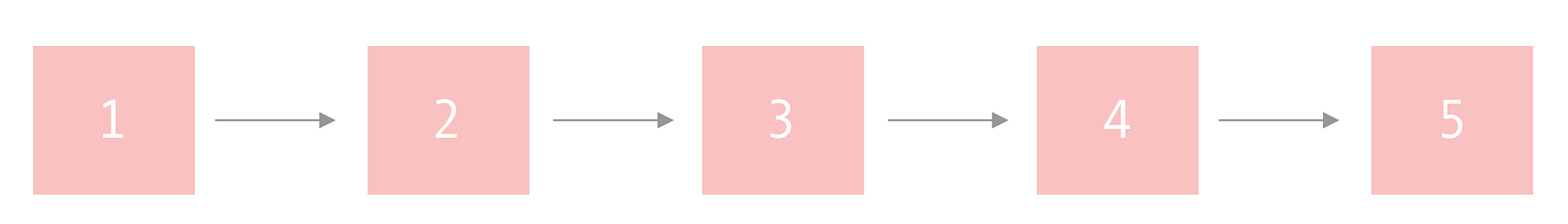Our node list will contain five methods:

• `push(value)`: Pushes a value on to the end of the linked list
• `pop()`: Pops off the last value from the list
• `get(index)`: Returns an item from a given index
• `delete(index)`: Deletes an item from a given index
• `isEmpty()`: Returns a boolean indicating whether the list is empty
• `printList()`: A method, not native to linked lists, which will print out our list; it’s primarily for debugging purposes

#### Constructor

I’m going to be using JavaScript class syntax, although you could also use a closure to create a linked list. So let’s set up the constructor.

We’ll need three pieces of information in our constructor:

• head: A reference to the node at the beginning of the list
• tail: A reference to the node at the end of the list
• length: How many nodes are in the list
``````class LinkedList {
constructor() {
this.tail = null;
this.length = 0;
}
}
``````

#### IsEmpty

The `isEmpty()` method is a helper function which returns true if the list is empty.

``````isEmpty() {
return this.length === 0;
}
``````

#### printList

This utility method will print the nodes in the list. This is solely meant for debugging purposes.

``````printList () {
const nodes = [];
while (current) {
nodes.push(current.value);
current = current.next;
}
return nodes.join(' -> ');
}
``````

#### Push

Our push method needs to check whether the list is empty or not before adding a new node. How do we know if the list is empty? Two ways:

• Our `isEmpty()` method returns true (the length of the list is zero)
• The head pointer is null

For this example, we’ll check whether head is null, although either solution works fine.

If there are no items in the list, we can simply set both the head and tail pointers to the new node and update the length of the list.

``````if (this.head === null) {
this.tail = node;
this.length++;
return node;
}
``````

If the list isn’t empty, we have to do the following:

• Set `tail.next` to point to the new node
• Set `tail` to point to the new node
• Increment the list length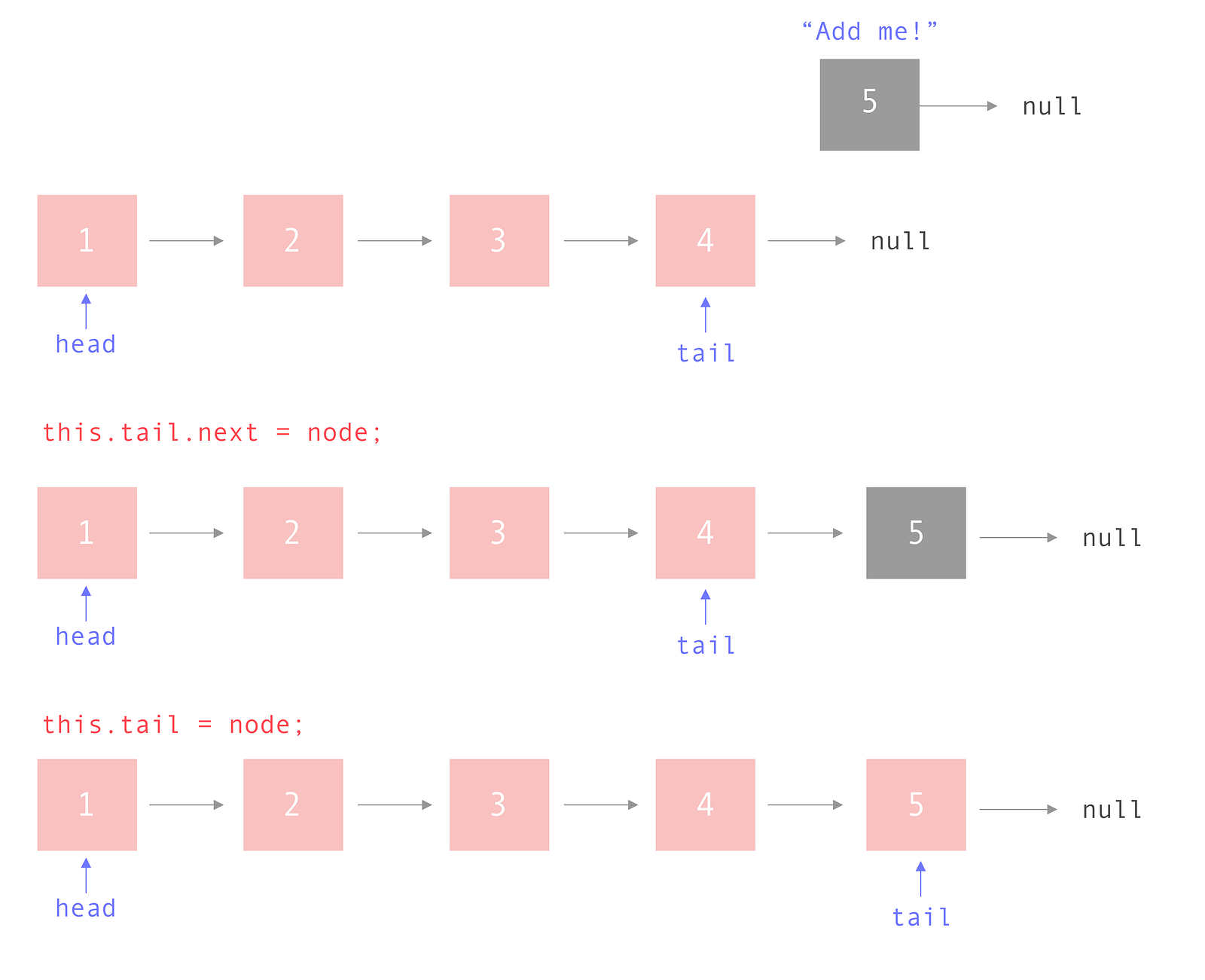Here is our completed push method:

``````push(value) {
const node = Node(value);
// The list is empty
this.tail = node;
this.length++;
return node;
}
this.tail.next = node;
this.tail = node;
this.length++;
}
``````

#### Pop

Our pop method needs check the following two things before removing the last item in the list:

• Check whether the list is empty
• Check whether there is only one item in the list

We can use our `isEmpty` method to check whether a list contains nodes.

``````if (this.isEmpty()) {
return null;
}
``````

How do we know if there’s only one node in the list? If head and tail are pointing to the same node. But what do we need to do in this instance? Removing the only node means we’re essentially resetting the list.

``````if (this.head === this.tail) {
this.tail = null;
this.length--;
return nodeToRemove;
}
``````

If there is more than one element in the list, we can do the following:

``````while there are nodes in the list
if the next node in the list is the tail
update tail to point to the current node
set the current node to point to null
decrement the length of the list
return the previous tail element
``````

It will look something like this:

``````let currentNode = this.head;
let secondToLastNode;

// Start at the front and iterate until
// we find the second to last node
while (currentNode) {
if (currentNode.next === this.tail) {
// Move the pointer for the second to last node
secondToLastNode = currentNode;
break;
}
currentNode = currentNode.next;
}
// Pop off that node
secondToLastNode.next = null;
// Move the tail to the second to last node
this.tail = secondToLastNode;
this.length--;

// Initialized to this.tail
return nodeToRemove;
``````

If you’re having trouble visualizing this, let’s walk through it.

Lines 6–10: If the next node in the list is the last item, this current item is the new “tail” so we need to save it’s reference.

``````if (currentNode.next === this.tail) {
secondToLastNode = currentNode;
}
``````

list

Line 15: Update `secondToLastNode` to point to null. This is the act of “popping” off the last element from the list.

``````secondToLastNode.next = null;
``````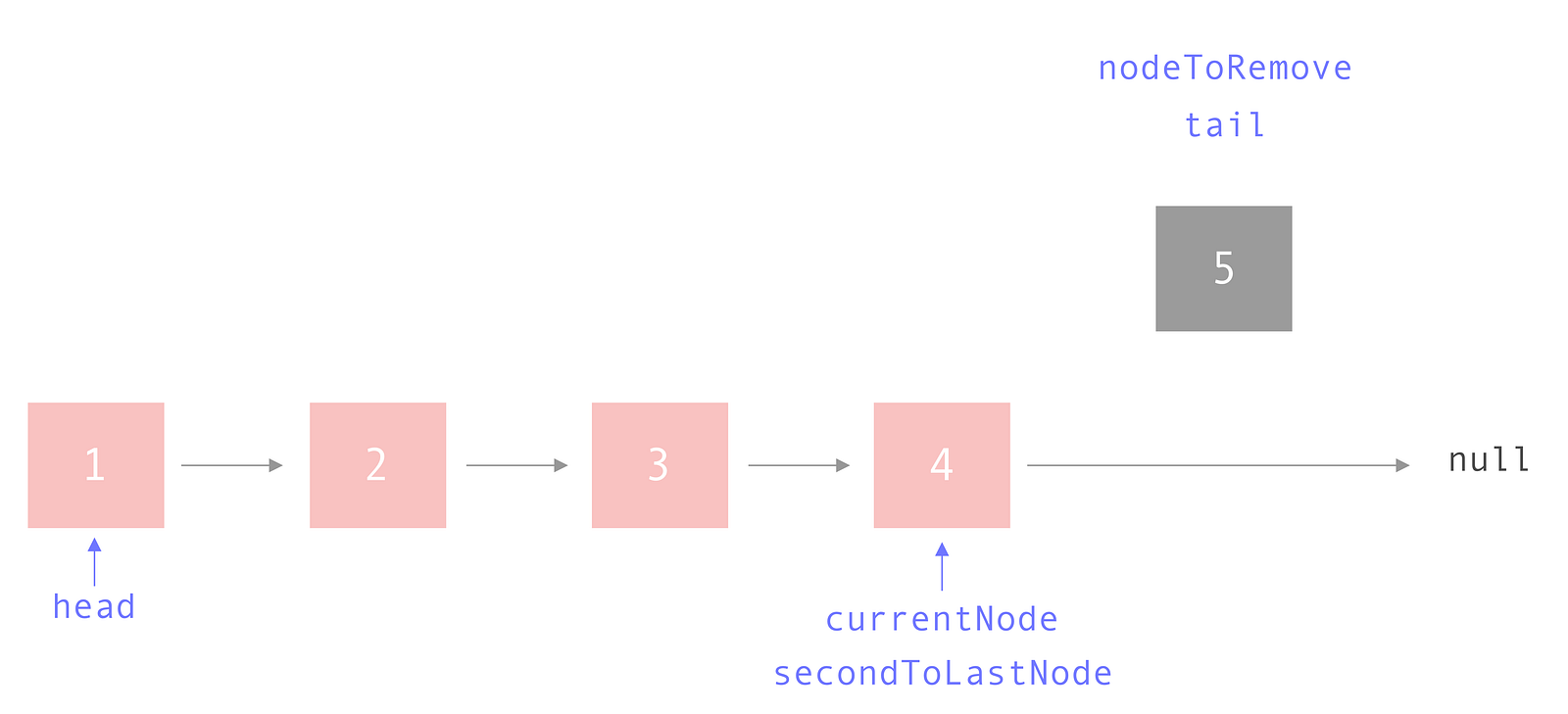Line 16: Update `tail` to point to `secondToLastNode`.

``````this.tail = secondToLastNode;
``````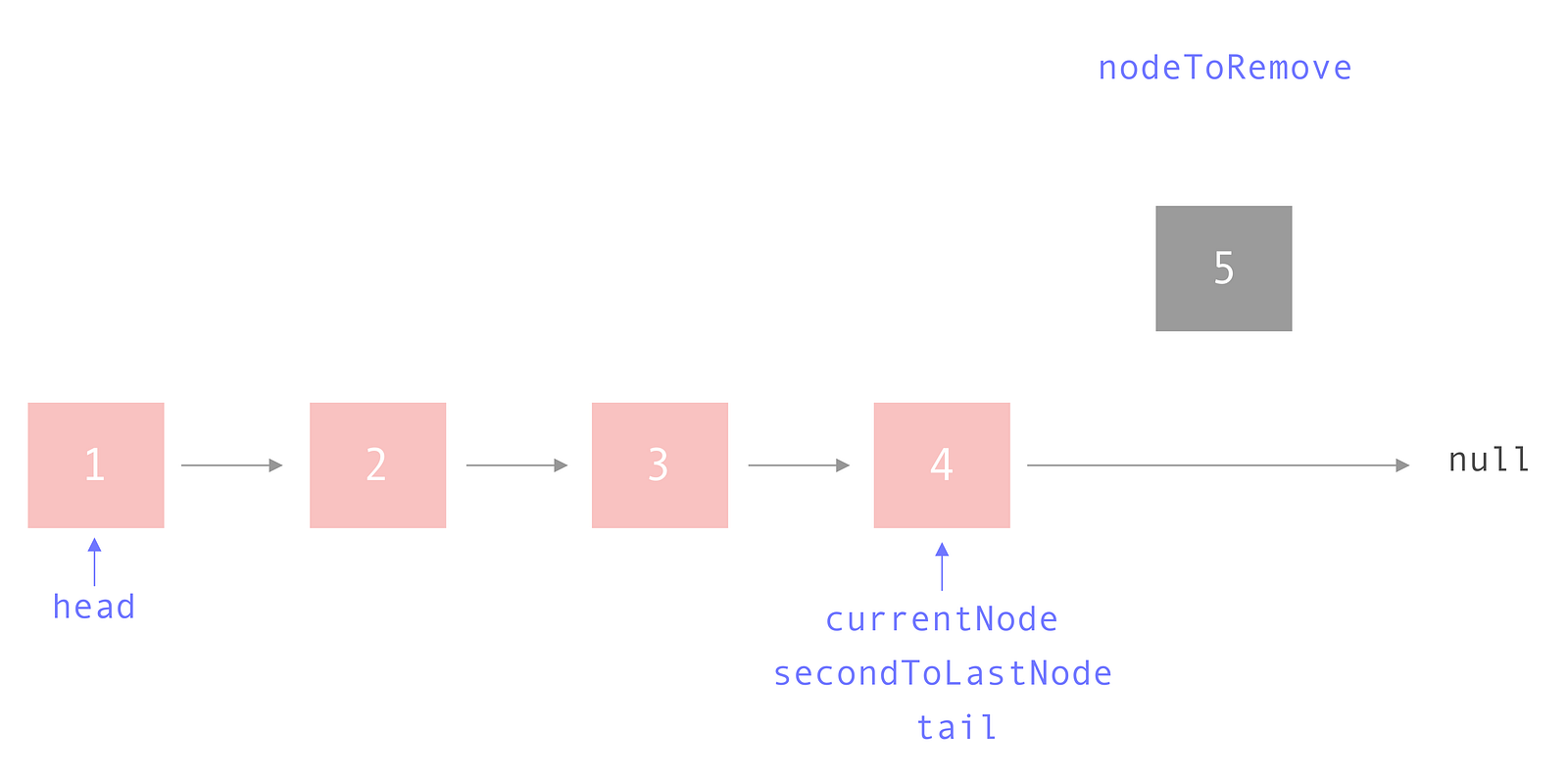Line 17: Decrement the length of the list because we just removed a node.

Line 18: Return the node we just popped off.

Here’s our full pop method:

``````pop() {
if (this.isEmpty()) {
return null;
}
const nodeToRemove = this.tail;
// There's only one node!
this.tail = null;
this.length--;
return nodeToRemove;
}

let secondToLastNode;

// Start at the front and iterate until
// we find the second to last node
while (currentNode) {
if (currentNode.next === this.tail) {
// Move the pointer for the second to last node
secondToLastNode = currentNode;
break;
}
currentNode = currentNode.next;
}
// Pop off that node
secondToLastNode.next = null;
// Move the tail to the second to last node
this.tail = secondToLastNode;
this.length--;

// Initialized to this.tail
return nodeToRemove;
}
``````

#### Get

Our get method must check for three situations:

• The index requested is outside the bounds of the list
• The list is empty
• We’re requesting the first element

If the requested index doesn’t exist within the list, return null.

``````// Index is outside the bounds of the list
if (index  this.length) {
return null;
}
``````

If the list is empty, return null. You can combine these if statements, but to keep it clear, I separated them.

``````if (this.isEmpty()) {
return null;
}
``````

If we’re requesting the first element, return the head.

``````// We're at the head!
if (index === 0 )  {
}
``````

Otherwise, we just iterate through the list one by one until we find the index we’re looking for.

``````let current = this.head;
let iterator =  0;

while (iterator < index) {
iterator++;
current = current.next;
}

return current;
``````

Here is the full `get(index)` method:

``````get(index) {
// Index is outside the bounds of the list
if (index  this.length) {
return null;
}

if (this.isEmpty()) {
return null;
}

if (index === 0 )  {
}

let iterator =  0;

while (iterator < index) {
iterator++;
current = current.next;
}

return current;
}
``````

#### Delete

Our delete method will also have to account for three special use cases:

• The index we want to delete is outside the bounds of the list
• The list is empty
• We want to delete the head

If the index we want to delete doesn’t exist within the list, return null.

``````// Index is outside the bounds of the list
if (index  this.length) {
return null;
}
``````

If the list is empty, return null. You could combine this logic with the logic to determine whether the index is outside the bounds of the list, but for clarity’s sake I have kept them separate.

``````if (this.isEmpty()) {
return null;
}
``````

If we want to delete the head, set `head` to the next value in the list, decrement the length, and return the value we just deleted.

``````if (index === 0) {
this.length--;
return nodeToDelete;
}
``````

If none of these booleans are true, the logic for deleting a node is as follows:

``````while the iterator isn't the index we're looking for
increase the iterator
move the previous and current pointers up by one
save the current value as the node to be deleted
update the previous node's pointer to point to the next node
if the next value is null
set tail to the new last node
decrement list length
return the deleted node
``````

If you need help visualizing this, please refer to the diagram found in the Pop section.

The difference between the delete method and the pop method is that the pop method will always delete the last item in the list. In contrast, the delete method can delete an index between 0 and the length of the list.

Here is the completed delete method:

``````delete(index) {
// Index is outside the bounds of the list
if (index  this.length - 1) {
return null;
}

if (this.isEmpty()) {
return null;
}

if (index === 0) {
this.length--;
return nodeToDelete;
}

let previous;
let iterator = 0;

while (iterator < index) {
iterator++;
previous = current;
current = current.next;
}
const nodeToDelete = current;
// Re-direct pointer to skip the element we're deleting
previous.next = current.next;

// We're at the end
if(previous.next === null) {
this.tail = previous;
}

this.length--;

return nodeToDelete;
}
``````

If you’d like to play around with the code, feel free to fork my CodePen:

See the Pen Linked List by Emma Wedekind (@emmawedekind) on CodePen.

## Creating Graphs With JavaScript

Graphs are a data structure comprised of a collection of nodes with edges. A graph can be directed or undirected.

A directed graph contains edges which function similar to a one-way street. The edge flows from one node to another.

For example, you might have a graph of people and movies where each person can have several favorite movies but movies do not have a favorite person.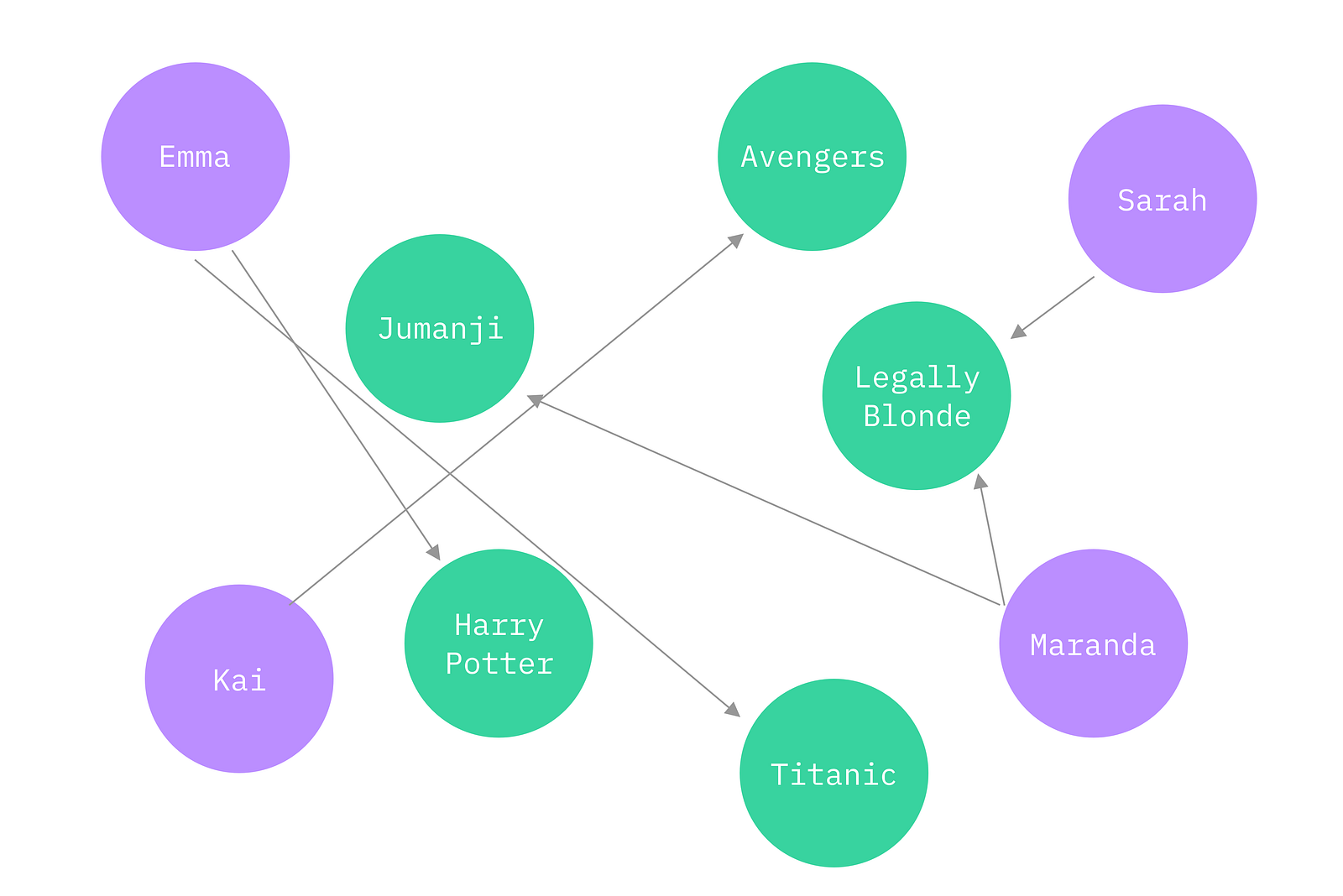An undirected graph contains edges which flow bi-directionally, similar to a two-lane road with traffic going in both directions.

For example, you might have a graph of pets where each pet has an owner and each owner has a pet. Note: The bi-directional arrows represent one edge, but for the sake of explicitness, I’ve drawn two arrows.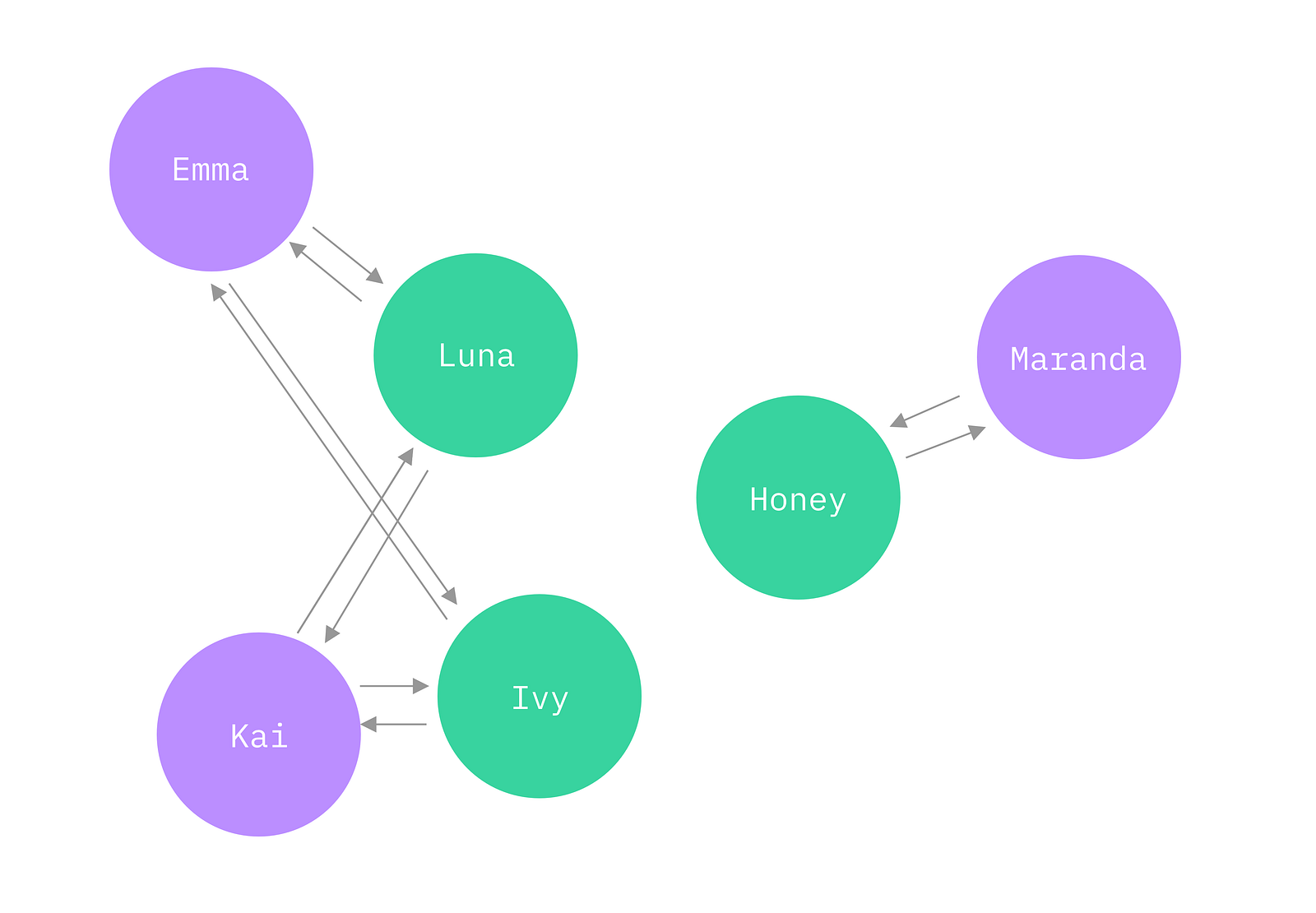There is no clear hierarchy of information in a graph.

### Methods

We’re going to build a graph of people and ice cream flavors. It will be a directed graph, as people can like certain flavors, but flavors do not like people.

We are going to create three classes:

• `PersonNode`
• `IceCreamFlavorNode`
• `Graph`

### PersonNode

The `PersonNode` class will take in one argument: a person’s name. This will serve as its identifier.

The `PersonNode` constructor will contain two properties:

• `name`: The unique identifier
• `favoriteFlavors`: An array of IceCreamFlavorNodes

Additionally, the `PersonNode` class will contain one method: `addFlavor`. This will take in one argument, an `IceCreamFlavorNode`, and add it to the `favoriteFlavors` array.

The class definition looks like this:

``````class PersonNode {
constructor(name) {
this.name = name;
this.favoriteFlavors = [];
}

this.favoriteFlavors.push(flavor);
}
}
``````

#### IceCreamFlavorNode

The `IceCreamFlavorNode` class will take in one argument: the ice cream flavor. This will serve as its identifier.

This class doesn’t need to contain any methods, as this is an undirected graph, with data flowing from the person to the flavors, but not backwards.

The class definition looks like this:

``````class IceCreamFlavorNode {
constructor(flavor) {
this.flavor = flavor;
}
}
``````

#### Graph

The `Graph` class won’t take in any arguments, but its constructor will contain three properties:

• `peopleNodes`: An array of PersonNodes.
• `iceCreamFlavorNodes`: An array of IceCreamFlavorNodes
• `edges`: An array containing the edges between PersonNodes and IceCreamFlavorNodes.

The Graph class will contain six methods:

• `addPersonNode(name)`: Takes in one argument, a person’s name, creates a new `PersonNode` with this name, and pushes it to the `peopleNodes` array.
• `addIceCreamFlavorNode(flavor)`: Takes in one argument, an ice cream flavor, creates a new `IceCreamFlavorNode` with this flavor, and pushes it to the `iceCreamFlavorNodes` array.
• `getPerson(name)`: Takes in one argument, a person’s name. and returns the node for that person.
• `getFlavor(flavor)`: Takes in one argument, an ice cream flavor. and returns the node for that flavor.
• `addEdge(personName, flavorName)`: Takes in two arguments, a person’s name and an ice cream flavor, retrieves both nodes, adds the flavor to the person’s `favoriteFlavors` array, and pushes the edge to the edges array.
• `print()`: Simply prints out each of the people in the `peopleNodes` array and their favorite ice cream flavors.

The class definition looks like this:

``````class Graph {
constructor() {
this.peopleNodes = [];
this.iceCreamFlavorNodes = [];
this.edges = [];
}

this.peopleNodes.push(new PersonNode(name));
}

this.iceCreamFlavorNodes.push(new IceCreamFlavorNode(flavor));
}

getPerson(name) {
return this.peopleNodes.find(person => person.name === name);
}

getFlavor(flavor) {
return this.iceCreamFlavorNodes.find(flavor => flavor === flavor);
}

const person = this.getPerson(personName);
const flavor = this.getFlavor(flavorName);
this.edges.push(`\${personName} - \${flavorName}`);
}

print() {
return this.peopleNodes.map(({ name, favoriteFlavors }) => {
return `\${name} => \${favoriteFlavors.map(flavor => `\${flavor.flavor},`).join(' ')}`;
}).join('\n')
}
}
``````

### Visualizing Data

Now that we have our three classes, we can add some data and test it out:

``````const graph = new Graph(true);

console.log(graph.print());
``````

Here’s what our directed graph looks like: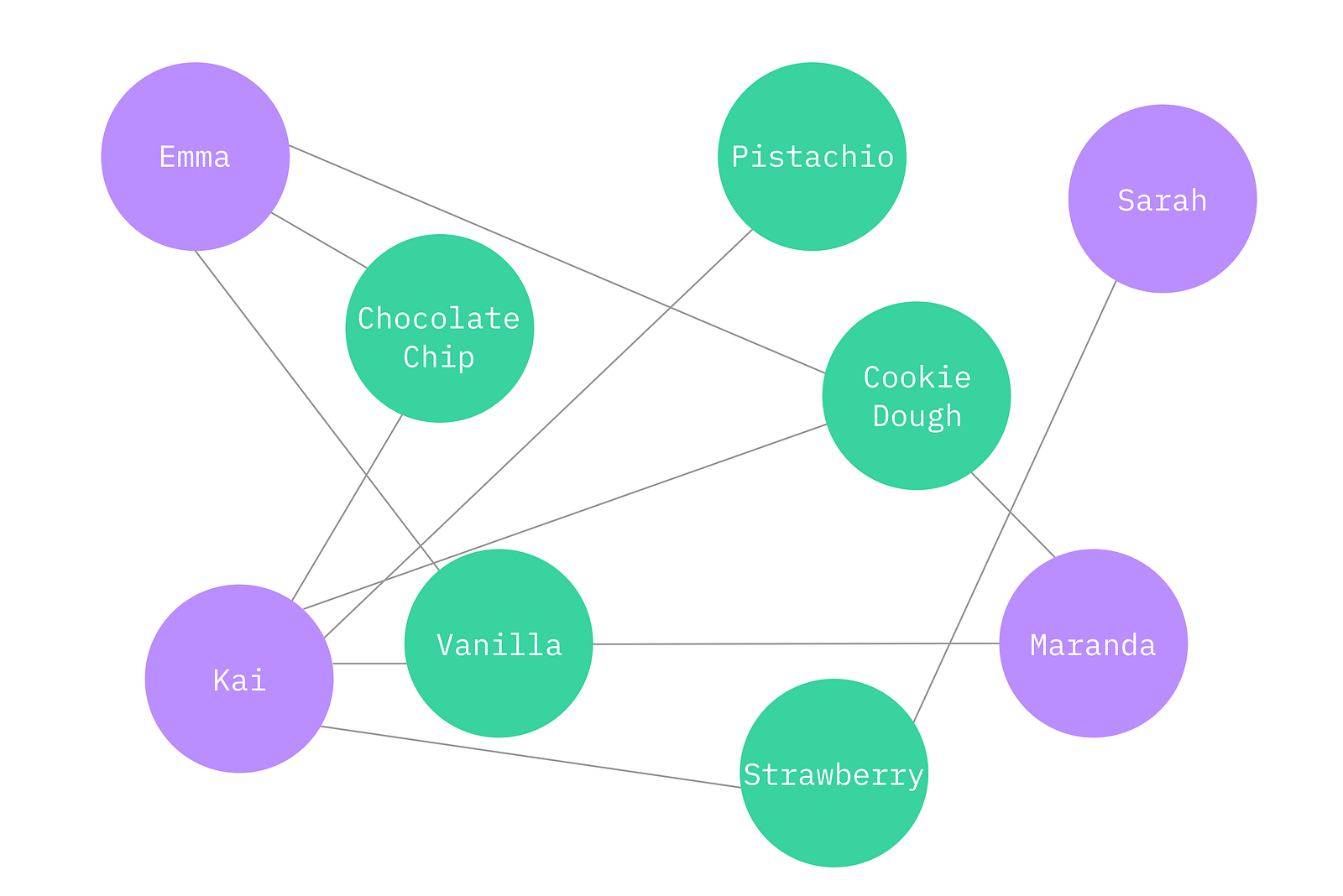If you’d like to see the code in its entirety, check out my CodePen:

See the Pen Graph by Emma Wedekind (@emmawedekind) on CodePen.

## Hire Web Developer

Looking for an attractive & user-friendly web developer? HourlyDeveloper.io, a leading web, and mobile app development company, offers web developers for hire through flexible engagement models. You can **[Hire Web...

With the rapid development in technology, the old ways to do business have changed completely. A lot more advanced and developed ways are ...

## A quick guide to JavaScript Arrays

6 most commonly used methods and how they’re implemented. Arrays are a structure common to all programming languages so knowing what they are and having a firm grasp on what you’re able to accomplish with Arrays will take you a long way in your journey as a software developer.